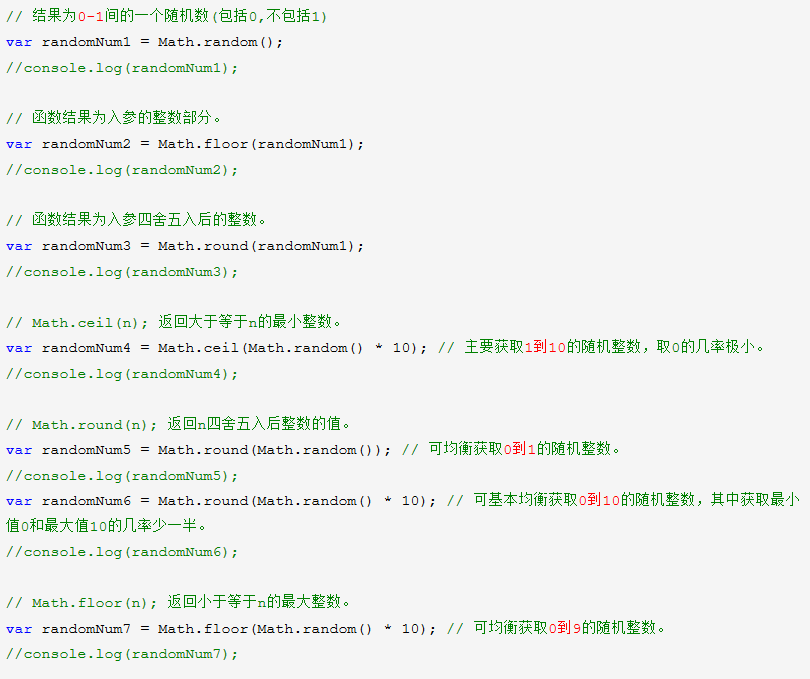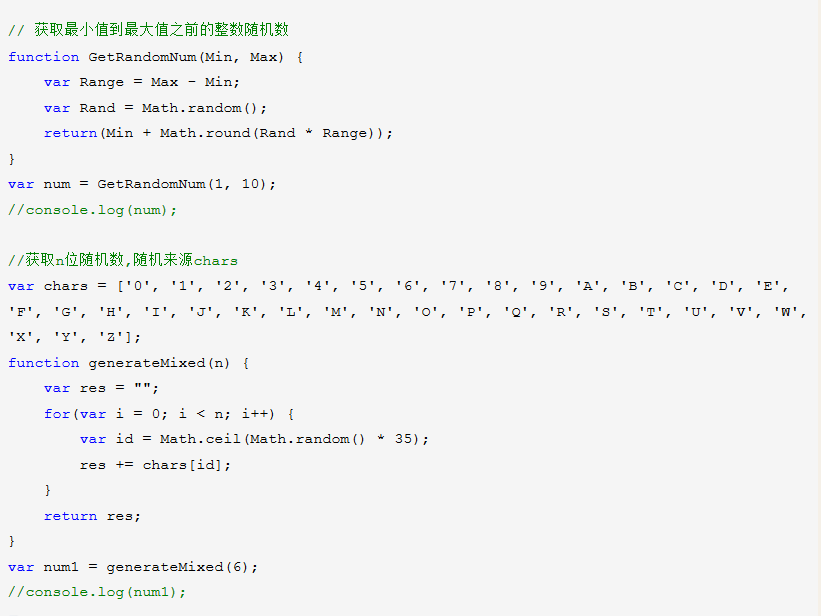• Js随机产生6为数字的代码，需要的朋友可以参考下。Js 随机数
•javascript
• javascript随机数和字符串生成库 用法： 从您的html页面使用它： [removed][removed] 或来自项目 // in your package.json dependencies: [ "random.js": "0.1.0" ] // in your js file var random = ...
• //获取随机范围内数值的函数 function GetRandom(n){GetRandomn=Math.floor(Math.random()*n+1)} //开始调用，获得一个1-100的随机数 GetRandom("100"); //输出查看 document.write(GetRandomn) GetRandomn就是...

方法1

复制代码 代码示例:

// 要创建一个随机浮点数时，使用rnd()；要创建一个随机整数时，例如，1到10时，使用rand(10)。

rnd.today=new Date();

rnd.seed=rnd.today.getTime();

function rnd() {

rnd.seed = (rnd.seed*9301+49297) % 233280;

return rnd.seed/(233280.0);

};

function rand(number) {

return Math.ceil(rnd()*number);

};

方法2：

复制代码 代码示例:

function selectFrom(iFirstValue,iLastValue)

{

var iChoces = Math.abs(iLastValue - iFirstValue)+1;

return Math.floor(Math.random() * iChoces + iFirstValue);

}

for(var i=0;i<50;i++){

}

方法3：

复制代码 代码示例:

//声明一个随机数变量，默认为1

var GetRandomn = 1;

//获取随机范围内数值的函数

function GetRandom(n){GetRandomn=Math.floor(Math.random()*n+1)}

//开始调用，获得一个1-100的随机数

GetRandom("100");

//输出查看

document.write(GetRandomn)

GetRandomn就是随机数的变量，可以任意调用了。

例子，随机显示。

复制代码 代码示例:

//现在开始，首先获得一个1到3的随机数

GetRandom("3");

来个范围的

例如 1-6

展开全文• 今天有又网友问到我 JavaScript 怎么生成指定范围数值随机数。Math.random() 这个方法相信大家都知道，是用来生成随机数的。不过一般的参考手册时却没有说明如何用这个方法来生成指定范围内的随机数。这次我就来详细...
• 主要为大家详细介绍了JavaScript实现随机数生成器，生成不重复的随机数，具有一定的参考价值，感兴趣的小伙伴们可以参考一下
• 这个时候我们就要知道用js应该如何获取一个随机数。一、js随机数知识Math.ceil(); //向上取整。Math.floor(); //向下取整。Math.round(); //四舍五入。Math.random(); //0.0 ~ 1.0 之间的一个伪随...

一、js随机数知识

Math.ceil();  //向上取整。

Math.floor();  //向下取整。

Math.round();  //四舍五入。

Math.random();  //0.0 ~ 1.0 之间的一个伪随机数。【包含0不包含1】 //比如0.8647578968666494

Math.ceil(Math.random()*10);      // 获取从1到10的随机整数 ，取0的概率极小。

Math.round(Math.random());   //可均衡获取0到1的随机整数。

Math.floor(Math.random()*10);  //可均衡获取0到9的随机整数。

Math.round(Math.random()*10);  //基本均衡获取0到10的随机整数，其中获取最小值0和最大值10的几率少一半。

因为结果在0~0.4 为0，0.5到1.4为1...8.5到9.4为9，9.5到9.9为10。所以头尾的分布区间只有其他数字的一半。

2.如果看不懂的话，也没关系，自己多写写demo然后回头再看这些属性，或者多做做项目，就会运用了！不要过于纠结这些，细枝末节！

3.生成随机数的公式！！

如果你希望生成任意值到任意值(也就是指定范围内)的随机数，我从网上找来了生成公式：Math.floor(Math.random()*(max-min+1)+min);

// max - 期望的最大值

// min - 期望的最小值

二 、js生成[n,m]的随机整数

函数功能：生成[n,m]的随机整数。

在js生成验证码或者随机选中一个选项时很有用。。

//生成从minNum到maxNum的随机数

functionrandomNum(minNum,maxNum){switch(arguments.length){case 1:return parseInt(Math.random()*minNum+1,10);break;case 2:return parseInt(Math.random()*(maxNum-minNum+1)+minNum,10);break;default:return 0;break;

}

}

js随机数生成过程分析：

Math.random()生成[0,1)的数，所以

Math.random()*5生成{0,5)的数。

通常期望得到整数，所以要对得到的结果处理一下。

parseInt()，Math.floor()，Math.ceil()和Math.round()都可得到整数。

parseInt()和Math.floor()结果都是向下取整。

所以Math.random()*5生成的都是[0,4] 的随机整数。

所以生成[1,max]的随机数，公式如下：

//max - 期望的最大值

parseInt(Math.random()*max,10)+1;

Math.floor(Math.random()*max)+1;

Math.ceil(Math.random()*max);

所以生成[0,max]到任意数的随机数，公式如下：

//max - 期望的最大值

parseInt(Math.random()*(max+1),10);

Math.floor(Math.random()*(max+1));

所以希望生成[min,max]的随机数，公式如下：

//max - 期望的最大值//min - 期望的最小值

parseInt(Math.random()*(max-min+1)+min,10);

Math.floor(Math.random()*(max-min+1)+min);

js随机数实例：js获取0-1之间的随机数，获取1-10之间的随机数

//获取0-1之间的随机数

var num = Math.random();

console.log(num);

//获取1-10之间的随机数

var num = Math.floor(Math.random() * 10 + 1);

console.log(num);

1)生成一个0 - 100 之间的随机整数，那么则可以：

parseInt(100*Math.random());

注意：因为Math.random()的返回值是包括0和1的，所以这里是有生成0和100的可能性的。

(2)生成一个从m - n 之间的随机整数，例如要生成一个 5 - 15 之间的随机数，则可以:

parseInt(Math.random()*(15-5+1) + 5);

概括即为：

parseInt(Math.random()*(n-m+1)+m);//生成一个从 m - n 之间的随机整数

另外根据需要总结了另外两种常用到的方法，如下：

(3)生成指定位数的随机整数

function randomNum(n){

var t='';

for(var i=0;i

t+=Math.floor(Math.random()*10);

}

return t;

}

(4)生成指定范围内的随机整数

function randomNum(minNum,maxNum){

switch(arguments.length){

case 1:

return parseInt(Math.random()*minNum+1);

break;

case 2:

return parseInt(Math.random()*(maxNum-minNum+1)+minNum);

break;

default:

return 0;

break;

}

}

例如生成 2 - 9之间的随机整数，则：randomNum(2,9)，生成 1 - 22之间随机整数，则：randomNum(22)

以上就是汇总的js生成随机数的方法，希望对大家的学习有所帮助。

展开全文• JS随机数

2019-08-12 01:24:10
NULL 博文链接：https://onestopweb.iteye.com/blog/2264138
• 转载于:https://www.cnblogs.com/gun-a/p/10176671.html转载于:https://www.cnblogs.com/gun-a/p/10176671.html

展开全文• js随机数基础 一、是javascript 随机数函数Math.random() random（Math.random 方法） public static random() : Number 返回一个伪随机数 n，其中 0 <= n < 1。返回的数字之所以称为”伪随机”数是因为它从...
• js随机数牵扯到的javascript的Math对象，Math提供对数据的数学计算，在随机数中常用的Math对象如下：1.Math.random(); 结果为0-1间的一个随机数(包括0,不包括1)2.Math.floor(num); 取结果的整数部分。3.Math.round...
• 本文实例讲述了JavaScript随机数的组合问题。分享给大家供大家参考，具体如下： 随机数的组合问题在面试时是经常考的，比如之前我就被问到：“有一个可以生成１－５的随机数函数，怎样把它扩大到１－７？” 在解决这...
• 3 主要使用了一下两个javascript函数 4 1、Math.floor() 方法可对一个数进行下舍入 5 2、Math.random() 方法可返回介于 0 ~ 1 之间的一个随机数 6 例如我们要取a-b区间的数字，并赋值给lanrenzhijia变量，则...
• js随机数

2019-11-28 11:23:01
单个自动随机数 //随机值= Math.floor(Math.random() * 可能值的总数 + 第一个可能的值) let random = Math.floor(Math.random() * 100 + 1); 两个数之间的随机数 function random(min, max) { if (min &...js随机数 随机数
• JS随机数不重复

千次阅读 2018-03-15 21:42:00
方法一思路：首先创建一个1到3000的数组，每次取一个数，然后去除数组中取出的这个数， 这样就可以实现永不重复。 var count=3000;var originalArray=new Array;//原数组//给原数组originalArray赋值for (var i=0;...
• 本文实例讲述了c#与js随机数生成方法。分享给大家供大家参考。具体如下： 1. C#产生随机数方法： 代码如下:Random rd = new Random(); rd.Next(low,high); 说明:生成70-100的随机数 代码如下:Random rd = new ...
• js中的随机数和小数取整 小数取整 (1)parseInt(5/2) 丢弃小数部分 保留整数 console.log(parseInt(5/2)); //====> 2 (2)Math.ceil(5/2) 向上取整，有小数就向上加一 (3)Math.floor(5/2) 向下取整 (4)Math.round...javascript
• JS随机数

2020-11-05 16:47:50
function randomNum(min, max) { if (min > max) { [min, max] = [max, min]; } let num = parseInt((max - min + 1) * Math.random() + min); return num; }javascript
• 有时候需要产生随机数。并让这些随机数出现的以概率的方式出现 下面举个例子： 随机产生1-8的整数， 希望 1的概率是50% 2的概率是10% 3的概率是10% 4的概率是10% 5的概率是5% 6的概率是5% 7的概率是5% 8的...java
• 基本思路：把Math.random()生成的数看着百分比，然后定义每个整数值取值范围。 具体内容如下，供大家参考 'use strict'; export default class GL { /** * 构造函数 * @param {object} opt ...
• 详细介绍了种子随机数JS中如何实现以及有什么作用，希望能帮助有需要的人。下面一起来看看。
• 一、是javascript 随机数函数Math.... [Ctrl+A 全选 注:如需引入外部Js需刷新才能执行]javascript 随机数 高级应用 ①自JavaScript产生后，好多浏览器中都有内置的随机数发生方法。例如： var number = Math.r
• js生成1到100的随机数 js生成随机数使用math.random()函数 Math.random() 具体实现： 1、定义一个random()函数，原理是 随机数和最大值减最小值的差相乘 最后再加上最小值。 function random(min, max) { return ...
• JS随机数、随机16位数、随机32位数、随机N位数 文章目录JS随机数、随机16位数、随机32位数、随机N位数前言一、随机数1.代码如下二、随机16位数、随机32位数、随机N位数1.代码如下总结 前言 提示：这里可以添加...js 小程序 javascript html5 css3...